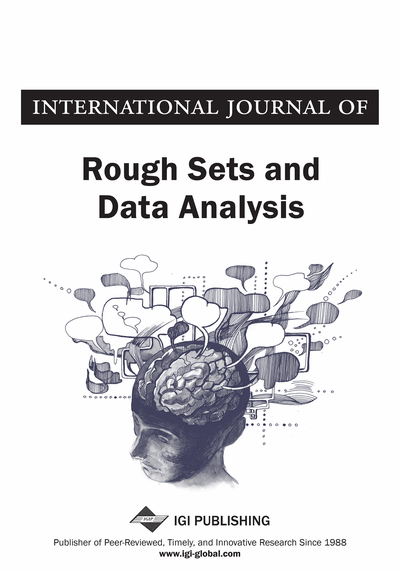# Algebraic Properties of Rough Set on Two Universal Sets based on Multigranulation

Mary A. Geetha (School of Computing Science and Engineering, VIT University, Vellore, Tamil Nadu, India), D. P. Acharjya (School of Computing Science and Engineering, VIT University, Vellore, Tamil Nadu, India) and N. Ch. S. N. Iyengar (School of Computing Science and Engineering, VIT University, Vellore, Tamil Nadu, India)
DOI: 10.4018/ijrsda.2014070104

## Abstract

The rough set philosophy is based on the concept that there is some information associated with each object of the universe. The set of all objects of the universe under consideration for particular discussion is considered as a universal set. So, there is a need to classify objects of the universe based on the indiscernibility relation (equivalence relation) among them. In the view of granular computing, rough set model is researched by single granulation. The granulation in general is carried out based on the equivalence relation defined over a universal set. It has been extended to multi-granular rough set model in which the set approximations are defined by using multiple equivalence relations on the universe simultaneously. But, in many real life scenarios, an information system establishes the relation with different universes. This gave the extension of multi-granulation rough set on single universal set to multi-granulation rough set on two universal sets. In this paper, we define multi-granulation rough set for two universal sets U and V. We study the algebraic properties that are interesting in the theory of multi-granular rough sets. This helps in describing and solving real life problems more accurately.
Article Preview
Top

## Introduction

The traditional concept of crisp sets has been extended in many directions as far as modeling of real life situations is concerned. The earliest is the notion of fuzzy set by l. A. Zadeh (1965) that captures impreciseness in information. On the other hand rough sets of z. Pawlak (1982, 1991) capture indiscernibility among objects to model imperfect knowledge. The basic philosophy is that human knowledge about a universe depends upon their capability to classify its objects. So, classification of a universe and indiscernibility relations defined on it are known to be interchangeable notions. The basic idea of rough set is based upon the approximation of sets by pair of sets known as lower approximation and upper approximation. Here, the lower and upper approximation operators are based on equivalence relations. However, the requirement of equivalent relation is a restrictive one and failure in many real life situations. In order to achieve this, rough set is generalized to binary relations (yao, (1998,2001,2004); kondo, 2006; pawlak & skowron, 2007a), fuzzy proximity relations (tripathy & acharjya (2008, 2010)), intuitionistic fuzzy proximity relations (tripathy, 2006; tripathy & acharjya (2009, 2011)), boolean algebras (liu, 2005; pawlak & skowron, 2007b), fuzzy lattices (liu, 2008), completely distributive lattices (chen et. Al., 2006) and neighborhood systems (lin, 1989). Development of these techniques and tools is studied under different domains like knowledge discovery in database, computational intelligence, knowledge representation, granular computing etc. (saleem durai et al., 2012; acharjya et al. (2011, 2012); tripathy et al., 2011).

In the view of granular computing, a general concept described by a set is always characterized by lower and upper approximations under static granulation. It indicates that the concept is depicted by means of single equivalence relation on the universe. However, in many real life situations, many concepts are described by using multi equivalence relations. Therefore, basic rough set model has been extended to rough set on multigranulations (qian et al., (2006, 2007)) in which the set approximations are defined by using multi-equivalences on the universe. On the other hand, rough set models on two universal sets are generalized with generalized approximation spaces and interval structure (wong et al., 1993). Here, the equivalence relation is generalized to binary relation.

## Complete Article List

Search this Journal:
Reset
Open Access Articles: Forthcoming
Volume 7: 4 Issues (2020): Forthcoming, Available for Pre-Order
Volume 6: 4 Issues (2019): 2 Released, 2 Forthcoming
Volume 5: 4 Issues (2018)
Volume 4: 4 Issues (2017)
Volume 3: 4 Issues (2016)
Volume 2: 2 Issues (2015)
Volume 1: 2 Issues (2014)
View Complete Journal Contents Listing